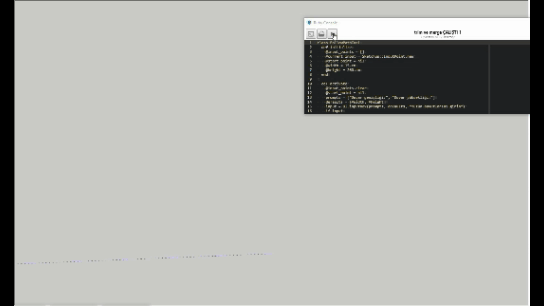# Is it possible to use "union" and "intersect_with" methods together?

Hello. This is a question about a problem I encountered in a script I prepared using “followme path” in Sketchup. I created a “merge” script that normally works properly and works as I want. But whenever the “followme reference lines” that I set the direction of intersect, a clean surface is not formed as in the “merge” process. The surfaces are intertwined as in the picture.
I created a code of my own to solve this problem. But this time the “merge” process did not work either. I wanted to ask you if i am on the right track. This is my code that works correctly, but after intersecting within itself, it is “nonsense”``````  class FollowPathRec
def initialize
@input_points = []
@current_input = Sketchup::InputPoint.new
@start_point = nil
end

def activate
@input_points.clear
@start_point = nil
end

def deactivate(view)
create_face if @input_points.length >= 2
view.invalidate
end

def onMouseMove(flags, x, y, view)
@current_input.pick(view, x, y)
view.invalidate
end

def onLButtonDown(flags, x, y, view)
@current_input.pick(view, x, y)
if @current_input.valid?
if @input_points.empty?
@start_point = @current_input.position
end
@input_points << @current_input.position
view.invalidate
end
end

CURSOR_PENCIL = 632
def onSetCursor
UI.set_cursor(CURSOR_PENCIL)
end

def onKeyUp(key, repeat, flags, view)
# I have defined here the tasks to be performed when I press the keys.
end

private

def create_face
# I created "siva" named groups with followme path.
end

def merge_groups
entities = Sketchup.active_model.entities
groups1 = entities.find_all { |e| e.is_a?(Sketchup::Group) && e.name == 'siva' }
merged_group1 = groups1.shift
groups1.each do |group|
intersection1 = merged_group1.bounds.intersect(group.bounds)
if intersection1.valid?
merged_group1 = merged_group1.union(group)

end
end
merged_group1.make_unique
merged_group1.name = 'siva'
end
end

model = Sketchup.active_model
follow_path_rec = FollowPathRec.new
Sketchup.active_model.select_tool(follow_path_rec)

``````

And this is the code fragment where I tried to use “union” and “intersect_with” methods at the same time.

``````def merge_groups
entities = Sketchup.active_model.entities
groups1 = entities.find_all { |e| e.is_a?(Sketchup::Group) && e.name == 'siva' }
merged_group1 = groups1.shift
groups1.each do |group|
intersection1 = merged_group1.bounds.intersect(group.bounds)
if intersection1.valid?
merged_group1 = merged_group1.union(group)

end
end

groups2 = entities.find_all { |e| e.is_a?(Sketchup::Group) && e.name == 'duvar' }
merged_group2 = groups2.shift
groups2.each do |group|
intersection2 = merged_group2.bounds.intersect(group.bounds)
if intersection2.valid?
merged_group2 = merged_group2.union(group)

end
end
merged_group2.make_unique
merged_group2.name = 'duvar'
merged_group1.make_unique
merged_group1.name = 'siva'
end
``````The solid operations will only work with groups and components that are solids.

There are two steps you will need to take to create a solid object from the geometry you’ve created.

First you’ll need to intersect all of the geometry in the group with itself.

``````model = Sketchup.active_model
grps = model.active_entities.grep( Sketchup::Group )
ents = grps.entities
ents.intersect_with(true, IDENTITY, ents, IDENTITY, true, ents.to_a)
``````

Secondly, loop through the edges and delete any edge that has three connected faces or that has two faces with the same surface normal. There may be other nontrivial cases that you’ll have to sort out .

``````model = Sketchup.active_model
grps = model.active_entities.grep( Sketchup::Group )
ents = grps.entities
edges = ents.grep( Sketchup::Edge )

togo = []
edges.each { | edge |
if edge.faces.size > 1 && edge.faces.size < 4
#p edge.faces.size
if edge.faces.size == 2
togo << edge if edge.faces.normal == edge.faces.normal
else
togo << edge
end
end
} if edges.size > 0

#p togo.size
ents.erase_entities( togo )
``````

and a test model with a non-solid group
A non-solid group.skp (105.5 KB)

1 Like

First use intersect_with
Then explode
Then grab all edges that are horizontal having 2 faces where each face has a plane that is horizontal.

There should be 8 edges which you can delete

1 Like

It really worked exactly the way I wanted it to. Thank you a lot`````` grps = model.active_entities.grep( Sketchup::Group )
grps.each do |grp|
if grp.name == "duvar"
ents = grp.entities
ents.intersect_with(true, IDENTITY, ents, IDENTITY, true, ents.to_a)
edges = ents.grep( Sketchup::Edge )
togo = []
edges.each { | edge |
if edge.faces.size > 1 && edge.faces.size < 4
#p edge.faces.size
if edge.faces.size == 2
togo << edge if edge.faces.normal == edge.faces.normal
else
togo << edge
end
end
} if edges.size > 0
end
ents.erase_entities( togo )
end
ents.intersect_with(true, IDENTITY, ents, IDENTITY, true, ents.to_a)
edges = ents.grep( Sketchup::Edge )
edges.each do |edge|
edge.erase! if edge.faces.size > 2
end
``````